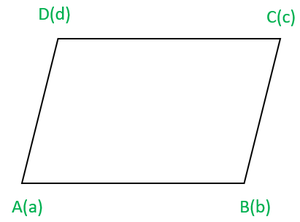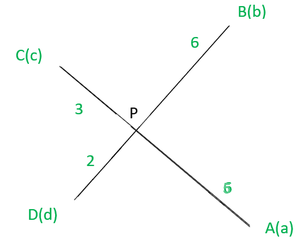Related Articles

# Class 12 RD Sharma Solutions – Chapter 23 Algebra of Vectors – Exercise 23.3

• Last Updated : 13 Jan, 2021

### Question 1. Find the position vector of a point R which divides the line joining the two points P and Q with position vectorsandrespectively in the ratio 1:2 internally and externally.

Solution:

The point R divides the line joining points P and Q in the ratio 1:2 internally.

The position vector of R =Point R divides the line joining P and Q in the ratio 1:2 externally.

The position vector of R =### Question 2. Letandbe the position vectors of the four distinct points A, B, C, D. Ifthen show that ABCD is a parallelogram.Solution:

Given that  are the position vectors of the four distinct points A, B, C, D

such thatGiven that,So, AB is parallel and equal to DC

Hence, ABCD is a parallelogram.

### Question 3.Ifare the position vectors of A, B respectively, find the position vector of a point C in AB produced such that AC = 3AB and that a point D in BA produced such that  BD = 2BA.

Solution:

Given thatare the position vector of A and B

Let C be a point in AB produced such that AC = 3AB.

From the given data we can say that point C divides the line AB in

Ratio 3:2 externally. So, the position vector of point C can be written as=D be a point in BA produced such that BD = 2BA

It is clear that point D divides the line in 1:2 externally.

Then the position vectorcan be written as=Henceand### Question 4.Show that the four points A, B, C, D with position vectorsandrespectively such thatare coplanar. Also, find the position vector of the point of intersection of the lines AC and BD.Solution:

Given thatSum of the coefficients on both sides of the given equation is 8

so, divide the equation by 8 on both the sidesIt is clear that the position vector of a point P dividing Ac in the

Ratio 3:5 is same as that of point P diving BD in the ratio 2:6.

Point P is common to AC and BD. Hence, P is the point of intersection of AC and BD.

Therefore, A, B, C and D are coplanar.

The position vector of point P can be written asor### Question 5: Show that the four points P, Q, R, S with position vectorsandrespectively such thatare coplanar. Also, find the position vector of the point of intersection of the lines PR and QS.

Solution:

Given thatHereandare the position vectors of point P, Q, R, S-(1)

Sum of the coefficients on both the sides of the equation (1) is 11.

So divide the equation (1) by 11 on both sides.It shows that position vector of a point A dividing PR in the ratio of 6:5 and

QS in the ratio 9:2. So A is the common point to PR and QS.

Therefore, P, Q, R and S are coplanar.

The position vector of point A is given byor### Question 6:The vertices A, B, C of triangle ABC have respectively position vectorswith respect to a given origin O. Show that the point D where the bisector ofmeets BC has position vectorwhere. Hence deduce that the incentre I has position vectorwhereSolution:

Let ABC be a triangle and the position vectors of A, B, C with respect to some origin say O be

Let D be the point on BC where the bisector ofmeets.be the position vector of D which divides BC internally in the ratioandwhereThus,Therefore, by section formula, the position vector of D is given byLetIncentre is the concurrent point of angle bisectors.

Thus, Incentre divides the line AD in the ratioand

the position vector of incentre is equal toAttention reader! All those who say programming isn’t for kids, just haven’t met the right mentors yet. Join the  Demo Class for First Step to Coding Coursespecifically designed for students of class 8 to 12.

The students will get to learn more about the world of programming in these free classes which will definitely help them in making a wise career choice in the future.

My Personal Notes arrow_drop_up